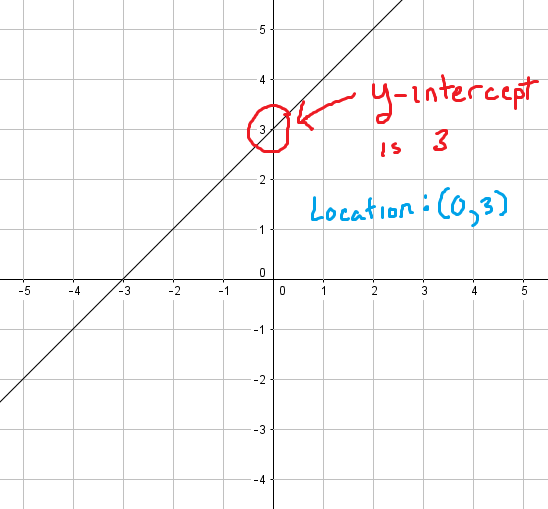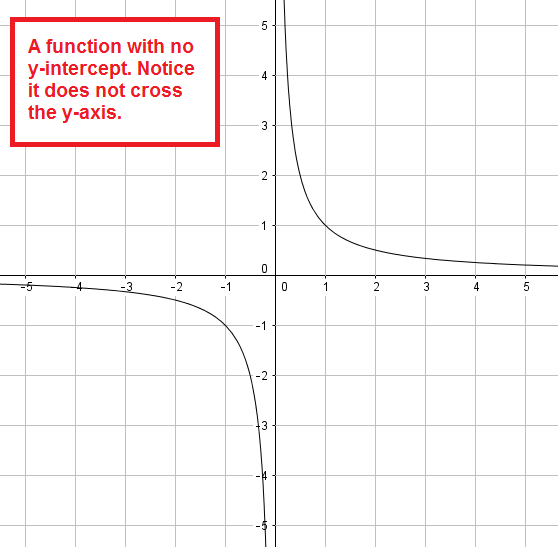﻿ Finding and understanding y-intercepts (with examples) - Math Bootcamps

# Understanding and finding y-intercepts

The y-intercept of a graph is the point where it crosses the y-axis, which is the vertical axis from the xy-coordinate plane. Below, we will see how to find the y-intercept of any function and why a function can have at most one y-intercept in general. You can also always scroll down to a video example.

## Seeing it on a graph

Before we go into detail, consider the graph below. As you can see, it is a linear function (the graph is a line) and it crosses the y-axis at the point (0, 3). This tells you that the y-intercept is 3.Since any point along the y-axis has an x-coordinate of 0, the form of any y-intercept is $$(0, c)$$ for some number $$c$$.

## Using algebra to find the y-intercept of a function

To find the y-intercept of a function, let $$x = 0$$ and solve for $$y$$. Consider the following example.

### Example

Find the y-intercept of the function: $$y = x^2 + 4x – 1$$

### Solution

Let $$x = 0$$ and solve for $$y$$.

\begin{align} y &= 0^2 + 4(0) – 1\\ &= \boxed{-1}\end{align}

Thus the y-intercept is –1 and is located at the point $$(0, –1)$$.

## A closer look

Now that we have seen how to find them, there are two interesting questions that can come up:

1. Can a function have more than one y intercept?
2. Can a function have no y intercept?

In answering these, remember that by definition, a function can only have one output (y-value) for each input (x-value). A function having more than one y-intercept would violate this, since it would mean that there are two outputs for $$x = 0$$. Therefore, it is not possible for a function to have more than one y-intercept.

What about no y intercept? Well, consider the graph below. This is a graph of the function: $$y = \dfrac{1}{x}$$This function never crosses the y-axis because, since you can’t divide by zero, it is undefined at $$x = 0$$. In fact, any time a function is undefined at 0, it will have no y-intercept.

## Video example

In the video below, I show you three examples of how to find the y-intercept. As you will see, the idea is pretty straight-forward!

## Summary

When working with any graph, two useful things to know are the location of any x-intercepts, and the location of the y-intercept, if it exists. With a linear function (a line) these two points are enough to quickly sketch a graph. For more complex functions however, finding intercepts is often part of a deeper analysis.

## Continue your study of graphing

You may find the following articles useful as you continue to study graphs: